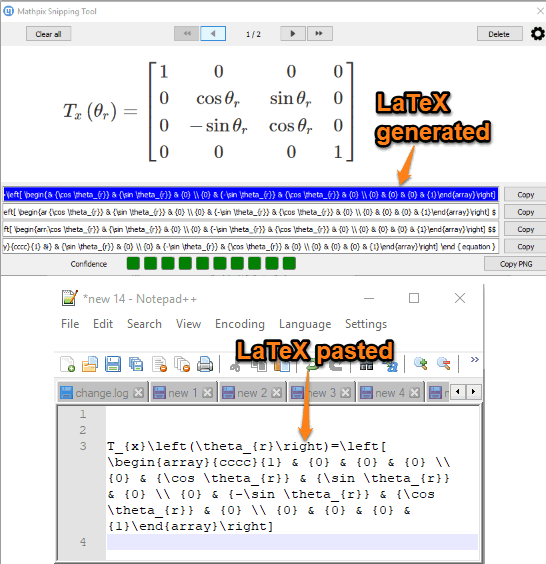Editor Ratings:
User Ratings:
[Total: 0 Average: 0]

This article covers how to convert Math equation available in an image to LaTeX. That simply means you can take the screenshot of the image containing Math equation and it will automatically be converted to LaTeX. Later, you can copy the output LaTeX and then paste it in an editor for further actions.

To convert a Math equation available in an image to LaTeX, I have covered a free and very simple software called “Mathpix“. It lets take a screenshot with a hotkey and then provides a separate window where that equation and output LaTeX is visible. You can also change hotkey and set the desired combination to create a new hotkey.In the screenshot above, you can see that a Matrix equation is converted to LaTeX which I further pasted in Notepad++.

## How to Convert Math Equation Available in an Image to LaTeX?

Step 1: Get the setup file with the help of the link given at the end of this article and install it. Launch the software and you’ll see a quick guide to help you use this software. You can follow that guide and then cancel that window.

Step 2: To capture a Math equation, use “Ctrl+Alt+M” hotkey, and select the area that covers the equation. You can change the hotkey by right-clicking its system tray icon and using the Settings option.

Step 3: As soon as the area is captured, a different window is opened. In that window, you will see that equation is visible and LaTeX output is also visible in different fields. There will also be a copy button for each LaTeX output.Use any button and copy LaTeX. Finally, you can paste that output to any text editor or LaTeX editor and then save it.

You may also read how to convert Math equations from plain text to Algebraic Expressions.

### The Conclusion:

Converting a Math equation available in an image to LaTeX is pretty simple and interesting with this software. Its OCR feature is quite good and it fetches the equation from image very well. You can give it a try.